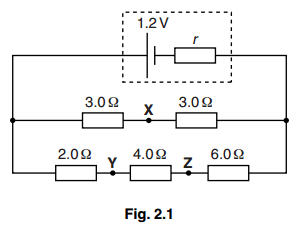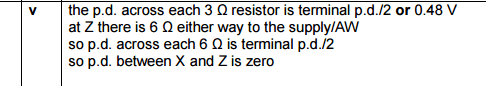# Potential difference between two points

## Homework StatementQuestion from OCR A Level Physics Exam 2014
(i) and (ii) = definitions
(iii) = explanation
(iv) = P.d. at 6Ohm Resistor = 0.48V

(v) Suggest why the p.d. between X and Z is zero

## Homework Equations

I don't think there are any

## The Attempt at a Solution

I couldn't understand the question at all so I looked up the answer in the mark scheme and I have no idea what it means.Could someone explain it to me?

Question Paper
Mark Scheme

cnh1995
Homework Helper
Gold Member
Welcome to PF!

## Homework Statement

View attachment 195271
Question from OCR A Level Physics Exam 2014
(i) and (ii) = definitions
(iii) = explanation
(iv) = P.d. at 6Ohm Resistor = 0.48V

(v) Suggest why the p.d. between X and Z is zero

## Homework Equations

I don't think there are any

## The Attempt at a Solution

I couldn't understand the question at all so I looked up the answer in the mark scheme and I have no idea what it means.
View attachment 195272
Could someone explain it to me?

Question Paper
Mark Scheme
You need to show your attempt. Per PF rules, members are not allowed to provide help here in the HH forums unless you show your working.
(iv) = P.d. at 6Ohm Resistor = 0.48V
Is that given?

Welcome to PF!

You need to show your attempt. Per PF rules, members are not allowed to provide help here in the HH forums unless you show your working.

Is that given?

No, it was not given:
(iii) asked why the current at X is twice current at Y/Z and I wrote because Total R at X loop = 6Ohms and Total R at Y/Z loop = 12Ohms therefore, since current is inversely proportional to resistance, current at X = 2 * current at Y/Z

I forgot to mention that it says current at point X is 0.16A so the current at Y/Z is 0.08A (since they already said X is double Y/Z)
(iv) asked what the p.d across the 6Ohm resistor was and by V=IR = 0.08 * 6 = 0.48V

I've been mulling over (v) for a while but I just couldn't understand what it asked at all.

cnh1995
Homework Helper
Gold Member
So are you clear with parts i) to iv) and you only need help with v)?

Look up 'balanced Wheatstone bridge'.

•LuTEX
So are you clear with parts i) to iv) and you only need help with v)?

Look up 'balanced Wheatstone bridge'.

Thanks! That seems to be it. So since the ratios of the resistances are equal, there is no p.d. in a bridge between those points, got it.
What I'm wondering now is why wasn't I taught this in class.

cnh1995
Homework Helper
Gold Member
What I'm wondering now is why wasn't I taught this in class.
You can analyse it using simple circuit laws.
Perhaps that's what was expected in this exercise rather than just using the bridge balance condition.
So since the ratios of the resistances are equal, there is no p.d. in a bridge between those points, got it.
That's a memory trick. I think you are supposed to prove it using circuit laws.

I think you are supposed to prove it using circuit laws.

Which laws would I use? Would it be that since both loops have equal total p.d.'s, the p.d. between them is zero (or something like that)?

epenguin
Homework Helper
Gold Member
You were helped by the question offering you a particularly simple example. The potential at X exactly halfway between those at the vertical wires , IOW the potential drop over the left top resistor is exactly the same as that in the right one because the two resistances are equal.
The same is true in the bottom branch because you have effectively two equal resistances of (2 + 4 =) 6Ω and 6Ω before and after Z.
For less simple cases this generalises to the proportionality considerations already mentioned (which you might work out considering any resistance of n Ω to be n resistors of 1Ω in series .

The potential at X, Z is not exactly 0.6 V because of the unknown resistance in series with the others.

The potential at X, Z is not exactly 0.6 V because of the unknown resistance in series with the others.
Where did you get 0.6 from?

epenguin
Homework Helper
Gold Member
Where did you get 0.6 from?

Half of 1.2 V, the battery. P.d. If r were 0 then the potential at X, Z would be 0.6 V. Witn r ≠ 0 it is not exactly this, but it is still exactly the same at X and Z.

Half of 1.2 V, the battery. P.d. If r were 0 then the potential at X, Z would be 0.6 V. Witn r ≠ 0 it is not exactly this, but it is still exactly the same at X and Z.
Ah yes. The question after this one asked to calculate r which I found to be 1 Ohm.

epenguin
Homework Helper
Gold Member
I also get 1 Ω .So I asked my teacher and they said they had actually forgotten to teach us how to do a question like this.